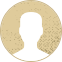Top Special Offer! Check discount

Get 13% off your first order - useTopStart13discount code now!

# Mean Math

286 views 3 pages ~ 682 words
Get a Custom Essay Writer Just For You!

Experts in this subject field are ready to write an original essay following your instructions to the dot!

## Calculate the arithmetic mean

An arithmetic mean is a statistical measurement of the central tendency of data. It is used in various academic disciplines. It is also known as the population mean. The average income of a nation's population is calculated by taking its arithmetic mean. This value is often used to compare countries in terms of their prosperity.

The arithmetic mean is a single value that best represents the distribution of a set of data. It is calculated by adding up the values in a dataset and dividing them by the total number of values (N). N is the number of samples or population. The arithmetic mean will be displayed if the calculation is successful.

The arithmetic mean is the most commonly used measure in descriptive statistics. It represents the average value of a characteristic across a sample. However, it is not a perfect indicator because outliers can affect the distribution. An outlier is an observation that is significantly different from the rest of the data. A large outlier will skew the average upwards, while a small outlier will push it down.

The arithmetic mean is also known as the equally-weighted average. It is derived by adding up the values of all the variables in a set. Then, the result is divided by the number of variables in the data set.

## Calculate the geometric mean

A geometric mean calculator is a tool used to find the geometric mean of a set of numbers. To use one, enter the numerical values, separated by commas or spaces, on a separate line. This tool will also calculate the standard deviation and variance. You can click on other measure options to get additional information, but these are not needed to calculate the geometric mean.

There are several applications of the geometric mean, including in medicine. In fact, it's considered the "gold standard" of measurement in some fields. It's also used in finance to calculate long-term investments and proportional growth. It is often used to estimate the risk-free rate for investments. Professor Aswath Damodoran, of NYU's corporate finance program, says the geometric mean is best used for long-term goals. In contrast, the arithmetic mean is more appropriate for short-term goals.

When you have a number set that has exponents that match, you can use the geometric mean to calculate its average. However, for computer languages, the log form is often used instead. The reason is that it's less likely to cause arithmetic overflow and underflow.

Another common application of the geometric mean is in finances, where it's useful for calculating average rates of return. A geometric mean can also help calculate growth over time. It's similar to the arithmetic mean, but takes the nth root of the values before multiplying them.

## Calculate the harmonic mean

A harmonic mean is a mathematical function that ranges from 0 to 1/2 for two different sets of numbers. It is also called a Schur-concave function. This means that the harmonic mean cannot be made arbitrarily large. This property makes it useful for arithmetic and geometric averages.

In a set of numbers, the harmonic mean always tends toward the lowest element, thus mitigating the impact of large or small outliers. In many cases, the arithmetic mean is confused with the harmonic mean, and is often used incorrectly in practice. A harmonic mean solver can be used to make sure you are using the right method.

To calculate the harmonic mean, you should divide the set of numbers by the reciprocal of each number. You must make sure that the numbers are positive and separate by commas or spaces. You should also make sure that you don't use the sample or population options when using the harmonic mean calculator. Once you've entered your numbers, the calculator will display your results and provide a link to additional calculations. If you'd rather use the mathematical formula, you can use Microsoft Excel to calculate the harmonic mean.

The harmonic mean is a mathematical concept commonly used in machine learning. It summarizes the most probable value for a variable. It is a useful tool in analyzing investment performance.

October 03, 2022
Category:
Subcategory:
Subject area:
Number of pages

3

Number of words

682

63Rate:

5

Expertise Mean
Verified writer

LuckyStrike has helped me with my English and grammar as I asked him for editing and proofreading tasks. When I need professional fixing of my papers, I contact my writer. A great writer who will make your writing perfect.

Hire Writer

This sample could have been used by your fellow student... Get your own unique essay on any topic and submit it by the deadline.

Eliminate the stress of Research and Writing!

Hire one of our experts to create a completely original paper even in 3 hours!